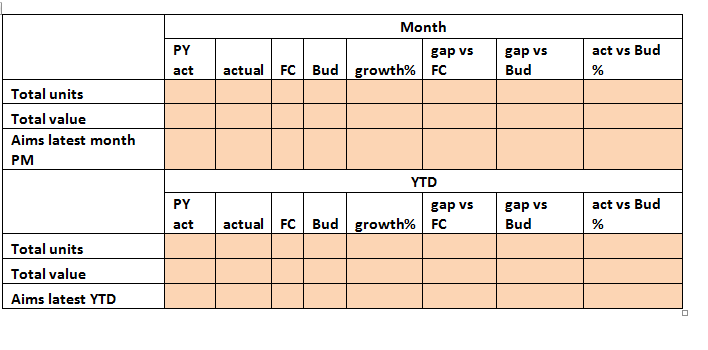# New to QlikView

Discussion board where members can get started with QlikView.

Contributor III

## How to get the YTD Chart correct and the "PY Actual" calculation correct?

Hi All ,

My Requirement is , I have to build report depending on the snapshot. Please verify if , my YTD chart is correct ?

My PY Actual expression is not working correctly .Please find the sample model attached.

1 Solution

Accepted SolutionsMVP

## Re: How to get the YTD Chart correct and the "PY Actual" calculation correct?

Hi,

The Max works with selection, so when you select 2015, the Max function will give 2015 as result and then the variable will give  2014 as result.

Regards,

Kaushik Solanki

7 RepliesMVP

## Re: How to get the YTD Chart correct and the "PY Actual" calculation correct?

Hi,

Change your vPrevFiscalYear variable to

=Max([Fiscal Year])-1

Regards,

Kaushik SolankiMVP

## Re: How to get the YTD Chart correct and the "PY Actual" calculation correct?

What you are trying for Current Year, As you said it's working good

Can you share the expression? And then, we might offer you to get PY

Before develop something, think If placed (The Right information | To the right people | At the Right time | In the Right place | With the Right context)
Contributor III

## Re: How to get the YTD Chart correct and the "PY Actual" calculation correct?

But , there is a possibility that I have to load for [Fiscal Year] 2014 until 2017 .

Thus , when I select 2015 , the report should work.MVP

## Re: How to get the YTD Chart correct and the "PY Actual" calculation correct?

Hi,

The Max works with selection, so when you select 2015, the Max function will give 2015 as result and then the variable will give  2014 as result.

Regards,

Kaushik Solanki

Contributor III

## Re: How to get the YTD Chart correct and the "PY Actual" calculation correct?

Sum(Aggr(if(Measure='Quantity',SUM({<[Fiscal Year]={\$(vPrevFiscalYear)},[Type Indicator] = {'A'} >} Quantity),if(Measure='Value',SUM({<[Fiscal Year]={\$(vPrevFiscalYear)},[Type Indicator] = {'A'} >} [Sales Value]),if(Measure='AIMS Units',SUM({<[Fiscal Year]={\$(vPrevFiscalYear)},[Type Indicator] = {'AIMS_SALES'} >} [Quantity]),SUM({<[Fiscal Year]={\$(vPrevFiscalYear)},[Type Indicator] = {'AIMS_SALES'} >} [Sales Value])))),Measure))

My CurrectYear is what, I select in the filter and PY should be Select Year -1.

Contributor III

## Re: How to get the YTD Chart correct and the "PY Actual" calculation correct?

Please can you verify , if my YTD Report expression are correct.MVP

## Re: How to get the YTD Chart correct and the "PY Actual" calculation correct?

What does this vPrevFiscalYear???

Before develop something, think If placed (The Right information | To the right people | At the Right time | In the Right place | With the Right context)